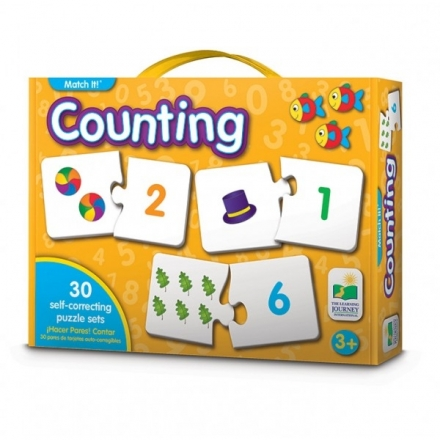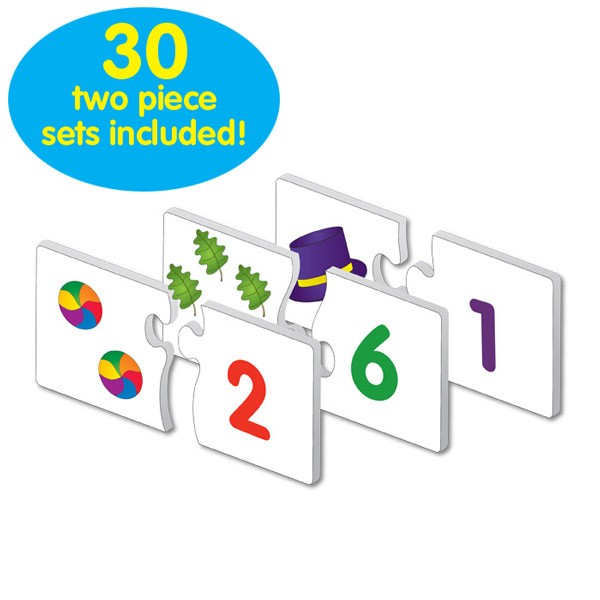Reduced price!# The Learning Journey MATCH IT! - COUNTING

New

TLJI-868942

### Introduction to mathematics and the concepts of number recognition and counting

• Ideal Age: 3y+
• 30 self-correcting puzzle pairs
• Teaches numbers 1 through 20

RM42.30

-10%

RM47.00

### MATCH IT! - COUNTING

This colorful set of 30 counting puzzle cards provides children with an excellent introduction to mathematics and the concepts of number recognition and counting . Each card features the number of pictures that are associated with the matching answer. Simply match the number the correct number of objects to complete the puzzle. The puzzles are self-correcting as no two puzzle cuts are the same. A fun way to learn counting.Features:

• This colorful set of 30 counting puzzle cards provides children with an excellent introduction to mathematics and the concepts of number recognition and counting
• Each card features the number of pictures that are associated with the matching answer
• Simply match the number the correct number of objects to complete the puzzle
• The puzzles are self-correcting as no two puzzle cuts are the same
• For ages 3 and up
• Includes 30 self-correcting puzzle pairs
• Teaches numbers 1 through 20
• Self-correcting puzzle pairs
• Colorful illustrations
• Builds self-confidence as children master skills